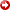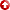--> Abstract: A Newton-Raphson Iterative Scheme for Integration of Multiphase Production Data into Reservoir Model, by Z. Wu; #90911 (2000)
[First Hit]A Newton-Raphson Iterative Scheme for Integration of Multiphase Production Data into Reservoir Model

WU, ZHAN, Texas A&M U.

The purpose of this paper is to present a new iterative inversion algorithm for integrating production data into geostatistical models. The significant feature of the proposed algorithm is that the computation of the sensitivity matrix for production data with respect to cell parameters can be avoided. This leads to dramatic reduction of computational cost. Generally, the computational cost of the gradient simulation method, adjoint method and Carter's method for generating sensitivity coefficient are dependent on the number of grid block, observed data and wells, respectively. In this paper, instead of generating the sensitivity matrix required for minimizing the least square objective function, our approach relies onsolvingthe inversionequationswhich are derived based on a necessary condition for a minimum. In comparison with other optimization methods, the proposed iteration scheme converges fast since the inversionequationscan be solved through the Newton-Raphson method. At each iteration, the computational requirement of our approach is to solve the finite differenceequationsand the linear adjointequationsonce, the reservoir parameters can be updated. Moreover, based on the Bayes theorem, geostatistical data can be honored simultaneously while the production data are matched.The proposed method is demonstrated for the inversion of permeability and porosity fields in a two-dimensional two-phase reservoir with nine production wells and four water injectors. Two numerical examples show that our new approach converges quickly for integrating the water oil ratio data into geostatistical models.

AAPG Search and Discovery Article #90911©2000 AAPG Pacific Section and Western Region Society of Petroleum Engineers, Long Beach, California